【一猫汽车网】“赤壁之山上摩空，三江之波浩无穷”。10月22日，由中国交通协会、赤壁人民政府联合东风汽车股份有限公司共同举办的东风凯普特·第二届中国卡友节油挑战赛物流专场比赛暨考察活动在美丽的三国古城湖北赤壁落下帷幕。中国交通运输协会物流技术装备专业委员会会长郭敏杰、中国交通运输协会物流技术装备委员会秘书长侍小彬、赤壁物流局局长胡文斌、东风轻型商用车销售有限公司总经理助理陈水敏等嘉宾共同出席。来自三家物流公司的卡友们因节油而聚集，与高效节油的东风轻卡一同开启全新挑战，用实际行动彰显了农村物流业发展的强大潜力。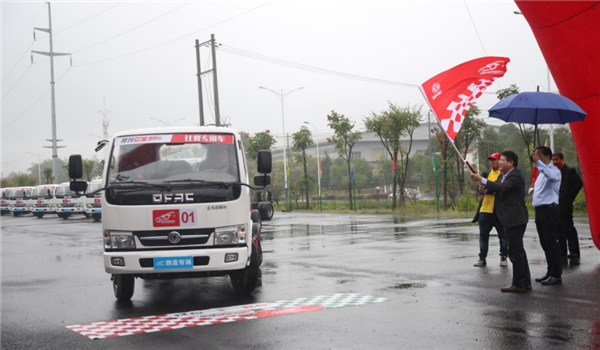“职业班子”间的较量  尤显精彩

细雨蒙蒙，为初秋清晨更添一份凉意。赛场上，来自赤壁市三家知名物流企业的参赛代表组成的20人方队整装待发。此次参赛选手们都是久经“沙场”的老手，也可以说是“职业司机”班子，实力自然不在话下。组团来参赛的卡友们，不仅是为个人荣誉而战，也是为团队荣誉而战。大家摩拳擦掌，等待着发令枪声的响起。随着主办方领导一个漂亮的挥旗，久经行业考验的卡友们驾驶着统一的比赛专用车——东风凯普特，纷纷亮出看家本领，踏上了冲锋之旅。

当天的小雨不仅没有影响选手们的发挥，反而让大家感受到更加真实的货运场景，体会到卡友们的艰辛，看到了他们应对困难、节油驾驶的强大实力。经过一上午的激战，两组选手陆续返回。工作人员进行了紧张有序的统计，成绩终于见分晓。来自中通的叶松波师傅摘得头魁，亚军和季军则分别落入来顺物流的龚成祖师傅和路路特团队的郭愿飞师傅囊中，三家物流团队均有斩获，足见职业班子实力的不分伯仲。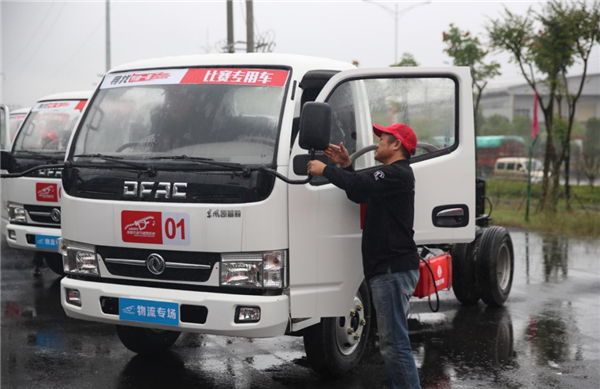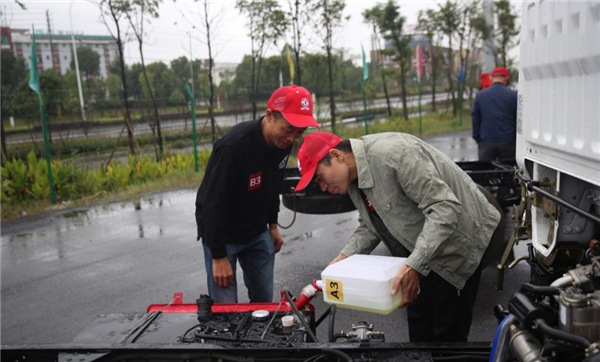同样的路况、同样的车型车况，最低油耗与最高油耗之间足足相差4.4L每百公里，这个油耗差距对于运输量巨大的物流企业而言无疑是非常值得注意的。现场，冠军得主资深司机叶师傅表示，“对于最终成绩还是有些意外的，我们一个团队的司机，日常驾驶中还没有形成足够的节油意识，认为大家的油耗应该差不多。通过比赛，我们直观的看到了油耗间的差距，如果人人都能节油，这对于公司来说无疑是节约了成本、提高了收入。我回去一定要向公司提议，多多举办内部的节油评比，让大家都养成科学节油驾驶的好习惯。”

通过比赛，通过一目了然的节油赛绩，无论是在场的专家、领导还是参赛用户，都明确了产品、驾驶方式以及科学的节油方式对物流业发展的巨大影响作用，并且更加坚定了节油发展的目标与方向。而这些差距引起的各方深思正让东风轻卡深觉不负此行，感受到使命达成的喜悦。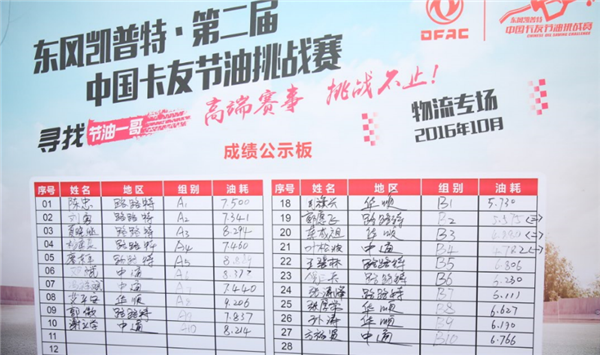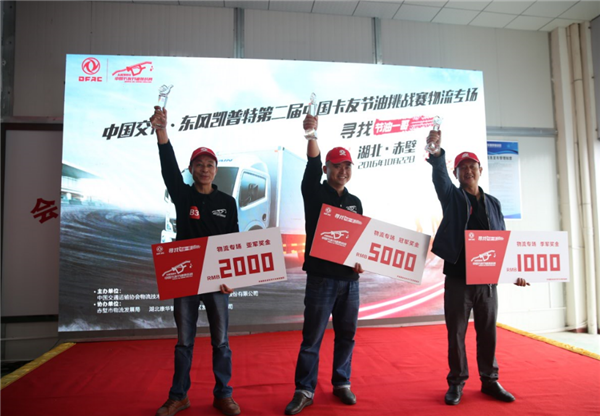本次物流专场，主办方设置的展览展示区也吸引了众多专家、领导及卡友的参观。节油高效的发动机、强劲可靠的好产品，都赢得了大家的赞誉。本次比赛的统一专用赛事车型东风凯普特E280也让卡友们交口称赞，其作为明星节油车型之一，优异的性能在今天的比赛中也充分得到了验证！东风凯普特E280车型基于国际化产品平台设计、生产，上市后以其超高的技术标准及产品品质，备受物流运输行业中高端用户的青睐。同时经历了2016年改款，凯普特E280在驾乘感受上也再上了一个新台阶。在2015年，东风旗下凯普特系列产品E280、N300、N280荣获第八届中国国际卡车节油大赛节油冠军及物流快递满意车型奖大奖，也成为了产品油耗全面超越其他品牌的有力鉴证！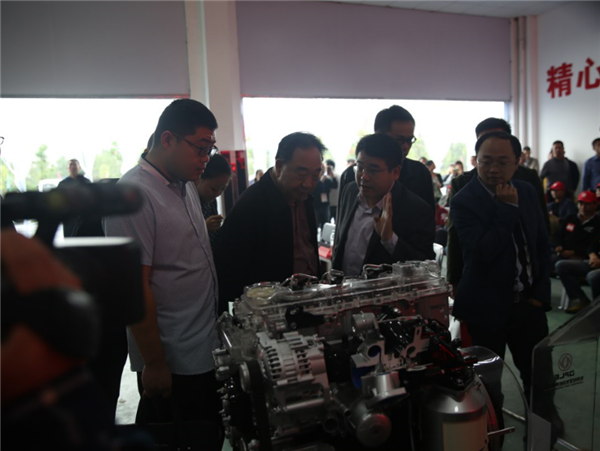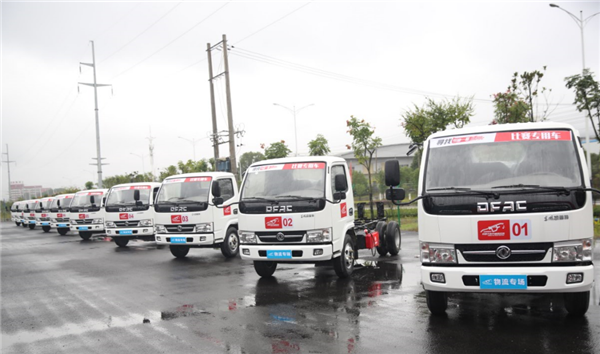对于举办此次物流专场的初衷，主办方领导表示，人和车，是物流行业所有模式的载体，也是物流行业与商用车企共同的交集。再好的物流模式，再先进的卡车装备，都需要职业的卡车司机去驾驭和营运，不管是物流车队，还是商用车企业，都应该重视卡车司机的技能提升，加快卡车司机的职业化进程。一个优秀的职业卡车司机跟一般司机比，油耗降低10%，事故率降低10%，都不是神话。对一个负责任的造车企业而言，研发一流的产品、正确的引导客户更好的驾驭产品，才能拥有更好的前景与未来。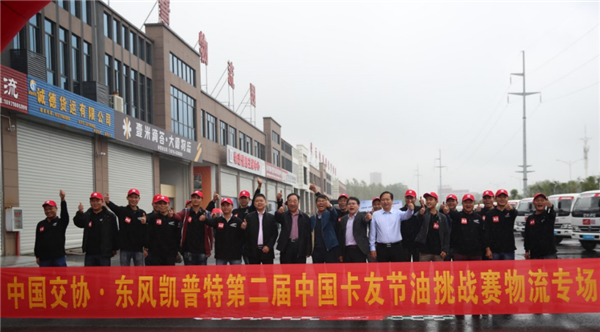东风汽车举办中国卡友节油挑战赛为不仅仅是为广大卡友们提供一个拼油技、互联动的展示平台，其积极创新增设物流专场也是助力高效物流运输、加速物流行业健康发展的一大举措。正如东风股份的领导在现场表示的一样，“我们希望借助赛事的深入推进，提升卡车司机节能降耗、文明驾驶的意识，加快卡车司机职业化进程；更希望通过绵薄之力推动物流运输行业乃至全社会节能降耗的深入践行、促进物流运输行业的发展、增强大众节能环保意识及带动整个社会效益的提升。”

##### 相关资讯

|分享

•已阅！握爪
•我手滑为你点赞
•128个赞！
•不明觉厉
•阅后既醉
•有钱！任性
•照片太美，我不敢看
•干货！杠杠的
•高大上
•膜拜中！
•请接受我的膝盖
•猫哥/猫妹么么哒
•神吐槽
•我只笑笑不说话
•我想静静
•窒息
##### 资讯相关车系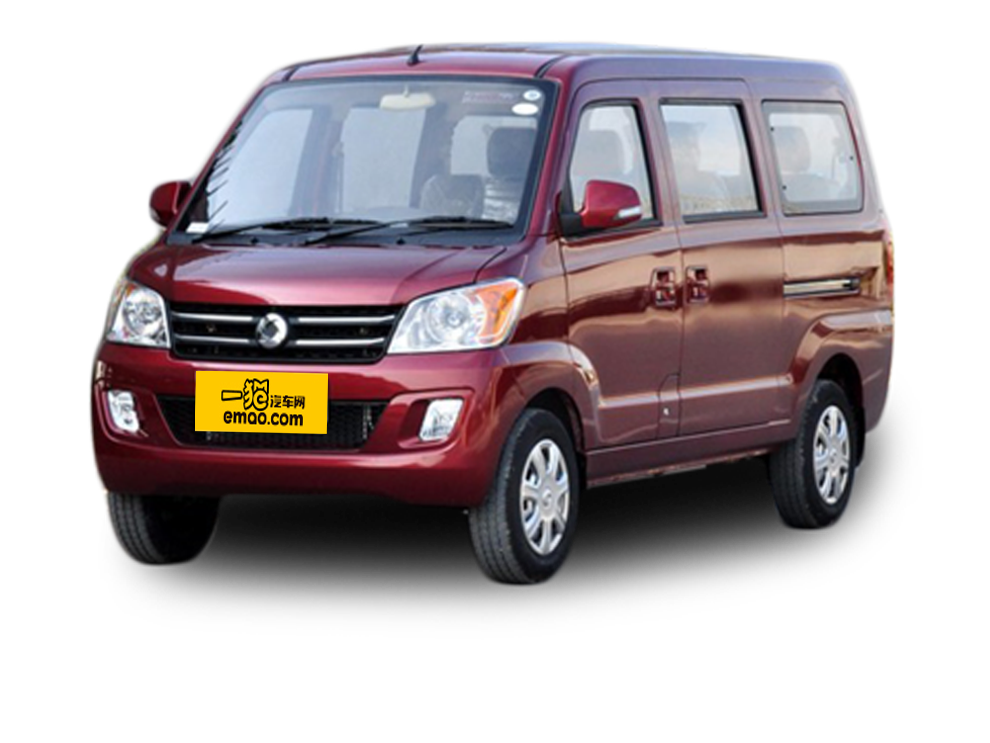##### 热度排行• 阿斯顿·马丁
• 奥迪
• 阿尔法罗密欧
• ALPINA

• 巴博斯
• 宝骏
• 宝马
• 保时捷
• 北汽制造
• 奔驰
• 奔腾
• 本田
• 比亚迪
• 标致
• 别克
• 宾利
• 布加迪
• 北汽威旺
• 北京
• 北汽绅宝
• 北汽幻速
• 北汽新能源
• 宝沃
• 比速汽车
• 北汽道达

• 昌河
• 长安
• 长城
• 长安商用
• 成功汽车
• 长江EV
• 长安轻车型

• 大众
• 道奇
• 东风
• 东风风神
• 东风小康
• 东南
• DS
• 东风风行
• 东风风度
• 东风风光

• 法拉利
• 菲亚特
• 丰田
• 福迪
• 福特
• 福田汽车
• 福汽启腾
• 风诺

• GMC
• 广汽传祺
• 广汽吉奥
• 观致

• 哈飞
• 海格
• 海马
• 华泰
• 黄海
• 恒天
• 红旗
• 哈弗
• 华颂
• 华凯
• 华泰新能源
• 汉腾汽车

• Jeep
• 江淮
• 江铃
• 捷豹
• 金杯
• 九龙
• 吉利汽车
• 金旅
• 金龙
• 江铃集团轻汽
• 江铃集团新能源
• 君马汽车
• 捷途

• 开瑞
• 凯迪拉克
• 科尼赛克
• 克莱斯勒
• KTM
• 卡威
• 凯翼
• 康迪
• 康迪电动汽车集团

• 猎豹汽车
• 兰博基尼
• 劳斯莱斯
• 雷克萨斯
• 雷诺
• 理念
• 力帆
• 莲花汽车
• 林肯
• 铃木
• 陆风
• 路虎
• 路特斯
• 领志
• 领克
• 零跑汽车

• MG
• MINI
• 马自达
• 玛莎拉蒂
• 迈凯伦
• 摩根

• 纳智捷

• 讴歌
• 欧宝
• 欧朗
• 欧拉
• 欧尚汽车

• 帕加尼

• 奇瑞
• 启辰
• 起亚
• 前途
• 庆铃汽车

• 日产
• 荣威
• 瑞麒汽车
• 如虎
• 瑞驰

• 上汽大通
• smart
• 三菱
• 双环
• 双龙
• 斯巴鲁
• 斯柯达
• 萨博
• 思铭
• 赛麟
• SWM斯威汽车

• TESLA
• 泰卡特
• 腾势

• 威麟
• 威兹曼
• 沃尔沃
• 五菱汽车
• 五十铃
• 潍柴英致
• WEY
• 蔚来
• 威马汽车

• 现代
• 雪佛兰
• 雪铁龙
• 西雅特
• 新特汽车
• 小鹏汽车
• 新宝骏

• 野马汽车
• 一汽
• 依维柯
• 英菲尼迪
• 永源
• 驭胜

• 中华
• 中兴
• 众泰
• 知豆
• 之诺
• 正道汽车
• A
• B
• C
• D
• E
• F
• G
• H
• I
• J
• K
• L
• M
• N
• O
• P
• Q
• R
• S
• T
• U
• V
• W
• X
• Y
• Z

• 阿斯顿·马丁
• 奥迪
• 阿尔法罗密欧
• ALPINA

• 巴博斯
• 宝骏
• 宝马
• 保时捷
• 北汽制造
• 奔驰
• 奔腾
• 本田
• 比亚迪
• 标致
• 别克
• 宾利
• 布加迪
• 北汽威旺
• 北京
• 北汽绅宝
• 北汽幻速
• 北汽新能源
• 宝沃
• 比速汽车
• 北汽道达

• 昌河
• 长安
• 长城
• 长安商用
• 成功汽车
• 长江EV
• 长安轻车型

• 大众
• 道奇
• 东风
• 东风风神
• 东风小康
• 东南
• DS
• 东风风行
• 东风风度
• 东风风光

• 法拉利
• 菲亚特
• 丰田
• 福迪
• 福特
• 福田汽车
• 福汽启腾
• 风诺

• GMC
• 广汽传祺
• 广汽吉奥
• 观致

• 哈飞
• 海格
• 海马
• 华泰
• 黄海
• 恒天
• 红旗
• 哈弗
• 华颂
• 华凯
• 华泰新能源
• 汉腾汽车

• Jeep
• 江淮
• 江铃
• 捷豹
• 金杯
• 九龙
• 吉利汽车
• 金旅
• 金龙
• 江铃集团轻汽
• 江铃集团新能源
• 君马汽车
• 捷途

• 开瑞
• 凯迪拉克
• 科尼赛克
• 克莱斯勒
• KTM
• 卡威
• 凯翼
• 康迪
• 康迪电动汽车集团

• 猎豹汽车
• 兰博基尼
• 劳斯莱斯
• 雷克萨斯
• 雷诺
• 理念
• 力帆
• 莲花汽车
• 林肯
• 铃木
• 陆风
• 路虎
• 路特斯
• 领志
• 领克
• 零跑汽车

• MG
• MINI
• 马自达
• 玛莎拉蒂
• 迈凯伦
• 摩根

• 纳智捷

• 讴歌
• 欧宝
• 欧朗
• 欧拉
• 欧尚汽车

• 帕加尼

• 奇瑞
• 启辰
• 起亚
• 前途
• 庆铃汽车

• 日产
• 荣威
• 瑞麒汽车
• 如虎
• 瑞驰

• 上汽大通
• smart
• 三菱
• 双环
• 双龙
• 斯巴鲁
• 斯柯达
• 萨博
• 思铭
• 赛麟
• SWM斯威汽车

• TESLA
• 泰卡特
• 腾势

• 威麟
• 威兹曼
• 沃尔沃
• 五菱汽车
• 五十铃
• 潍柴英致
• WEY
• 蔚来
• 威马汽车

• 现代
• 雪佛兰
• 雪铁龙
• 西雅特
• 新特汽车
• 小鹏汽车
• 新宝骏

• 野马汽车
• 一汽
• 依维柯
• 英菲尼迪
• 永源
• 驭胜

• 中华
• 中兴
• 众泰
• 知豆
• 之诺
• 正道汽车
• A
• B
• C
• D
• E
• F
• G
• H
• I
• J
• K
• L
• M
• N
• O
• P
• Q
• R
• S
• T
• U
• V
• W
• X
• Y
• Z

• 阿斯顿·马丁
• 奥迪
• 阿尔法罗密欧
• ALPINA

• 巴博斯
• 宝骏
• 宝马
• 保时捷
• 北汽制造
• 奔驰
• 奔腾
• 本田
• 比亚迪
• 标致
• 别克
• 宾利
• 布加迪
• 北汽威旺
• 北京
• 北汽绅宝
• 北汽幻速
• 北汽新能源
• 宝沃
• 比速汽车
• 北汽道达

• 昌河
• 长安
• 长城
• 长安商用
• 成功汽车
• 长江EV
• 长安轻车型

• 大众
• 道奇
• 东风
• 东风风神
• 东风小康
• 东南
• DS
• 东风风行
• 东风风度
• 东风风光

• 法拉利
• 菲亚特
• 丰田
• 福迪
• 福特
• 福田汽车
• 福汽启腾
• 风诺

• GMC
• 广汽传祺
• 广汽吉奥
• 观致

• 哈飞
• 海格
• 海马
• 华泰
• 黄海
• 恒天
• 红旗
• 哈弗
• 华颂
• 华凯
• 华泰新能源
• 汉腾汽车

• Jeep
• 江淮
• 江铃
• 捷豹
• 金杯
• 九龙
• 吉利汽车
• 金旅
• 金龙
• 江铃集团轻汽
• 江铃集团新能源
• 君马汽车
• 捷途

• 开瑞
• 凯迪拉克
• 科尼赛克
• 克莱斯勒
• KTM
• 卡威
• 凯翼
• 康迪
• 康迪电动汽车集团

• 猎豹汽车
• 兰博基尼
• 劳斯莱斯
• 雷克萨斯
• 雷诺
• 理念
• 力帆
• 莲花汽车
• 林肯
• 铃木
• 陆风
• 路虎
• 路特斯
• 领志
• 领克
• 零跑汽车

• MG
• MINI
• 马自达
• 玛莎拉蒂
• 迈凯伦
• 摩根

• 纳智捷

• 讴歌
• 欧宝
• 欧朗
• 欧拉
• 欧尚汽车

• 帕加尼

• 奇瑞
• 启辰
• 起亚
• 前途
• 庆铃汽车

• 日产
• 荣威
• 瑞麒汽车
• 如虎
• 瑞驰

• 上汽大通
• smart
• 三菱
• 双环
• 双龙
• 斯巴鲁
• 斯柯达
• 萨博
• 思铭
• 赛麟
• SWM斯威汽车

• TESLA
• 泰卡特
• 腾势

• 威麟
• 威兹曼
• 沃尔沃
• 五菱汽车
• 五十铃
• 潍柴英致
• WEY
• 蔚来
• 威马汽车

• 现代
• 雪佛兰
• 雪铁龙
• 西雅特
• 新特汽车
• 小鹏汽车
• 新宝骏

• 野马汽车
• 一汽
• 依维柯
• 英菲尼迪
• 永源
• 驭胜

• 中华
• 中兴
• 众泰
• 知豆
• 之诺
• 正道汽车
• A
• B
• C
• D
• E
• F
• G
• H
• I
• J
• K
• L
• M
• N
• O
• P
• Q
• R
• S
• T
• U
• V
• W
• X
• Y
• Z